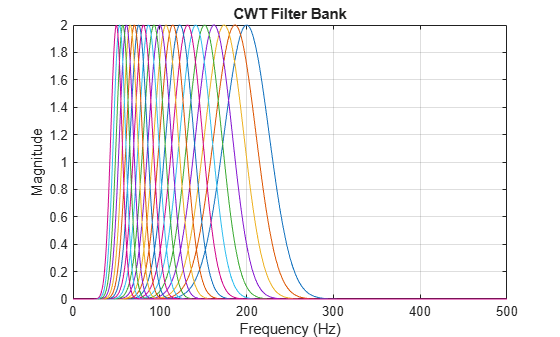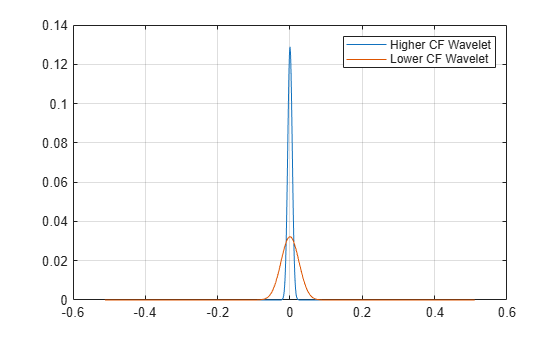Documentation

wavelets

CWT filter bank time-domain wavelets

Description

psi = wavelets(fb) returns the time-domain wavelets psi for the continuous wavelet transform (CWT) filter bank fb. The time-domain wavelets are centered at the origin.

example

[psi,t] = wavelets(fb) returns the sampling instants t for the wavelets.

Examples

collapse all

Create a continuous wavelet transform filter bank. Set the sampling frequency to 1000 Hz and the frequency limits to range from 50 Hz to 200 Hz. Plot the frequency response.

fb = cwtfilterbank('SamplingFrequency',1000,'FrequencyLimits',[50 200]);
freqz(fb)Obtain the filter bank time-domain wavelets. Plot the magnitudes of the first and last wavelets contained in the output. The first wavelet corresponds to the wavelet filter with center frequency equal to 200 Hz, and the last wavelet corresponds to the wavelet filter with center frequency equal to 50 Hz.

[psi,t] = wavelets(fb);
figure
plot(t,abs(psi(1,:)))
hold on
plot(t,abs(psi(end,:)))
legend('Higher CF Wavelet','Lower CF Wavelet')
grid onInput Arguments

collapse all

Continuous wavelet transform (CWT) filter bank, specified as a cwtfilterbank object.

Output Arguments

collapse all

Time-domain wavelets, returned as a Ns-by-N complex-valued matrix, where Ns is the number of wavelet bandpass frequencies (equal to the number of scales) and N is the filter bank SignalLength. The wavelets are ordered in psi from the highest-frequency passband filter to the lowest-frequency passband filter.

Sampling instants of the time-domain wavelets, returned as a real-valued vector of length N, where N is the filter bank SignalLength. The data type of t is the same as the SamplingPeriod.